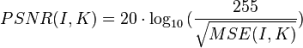# PSNR

PSNR stands for Peak Signal-to-Noise Ratio. It is used as a measure of video quality. It is expressed in decibels. It's defined aswhere I is the reference image, K is the image under test, and MSE is the Mean Squared Error between the two:

Where M is the number of pixels in a frame (width · height)

The double-Σ term states that (j,k) runs over all the pixels, summing the square of the difference between reference image I and test image K.